Examples

Chapter 11 Class 11 Conic Sections
Serial order wise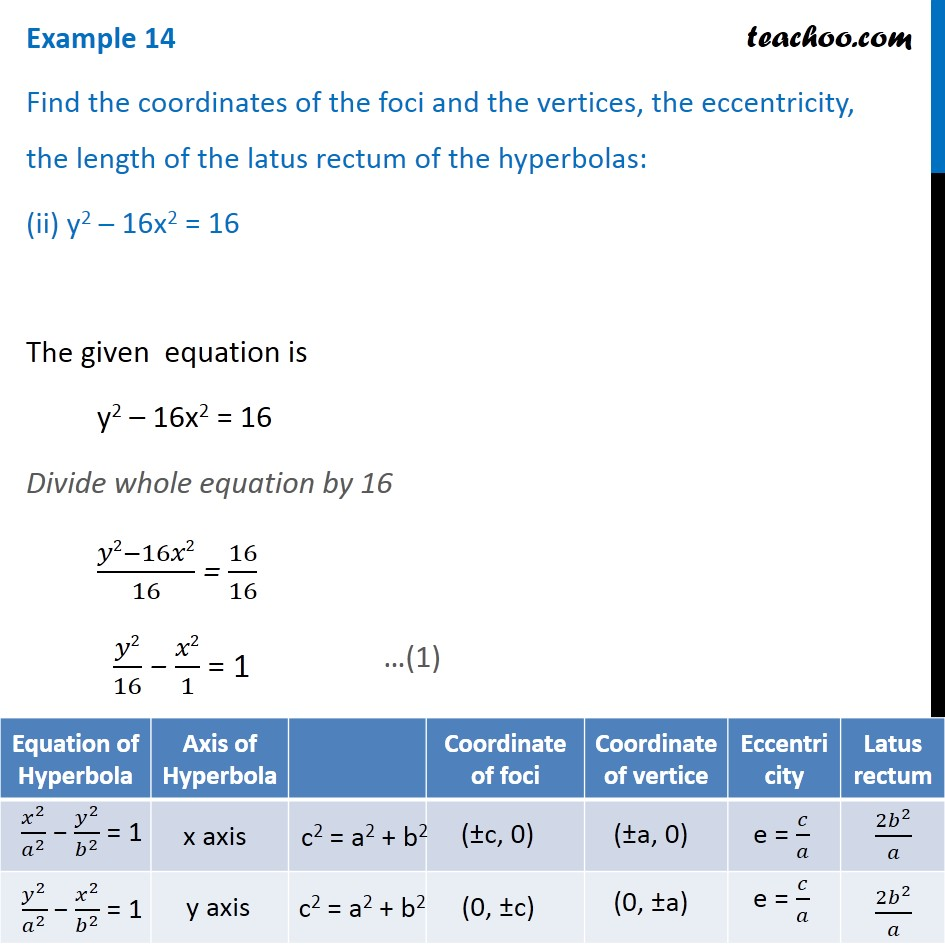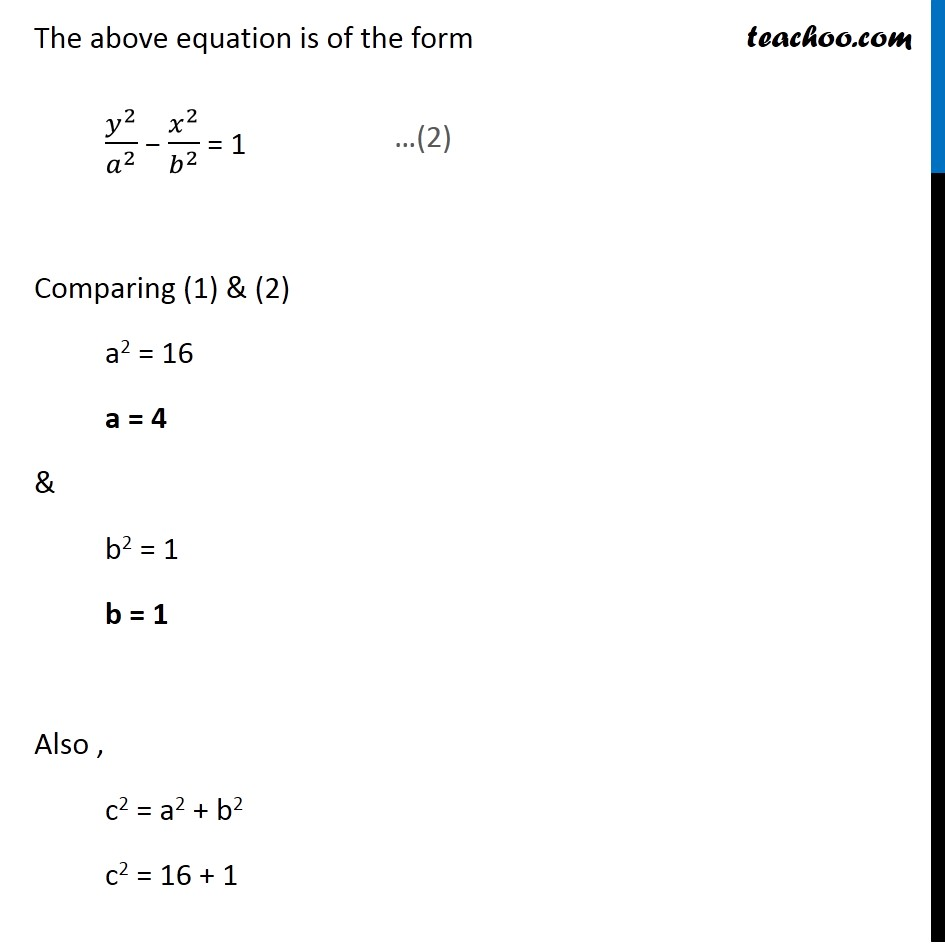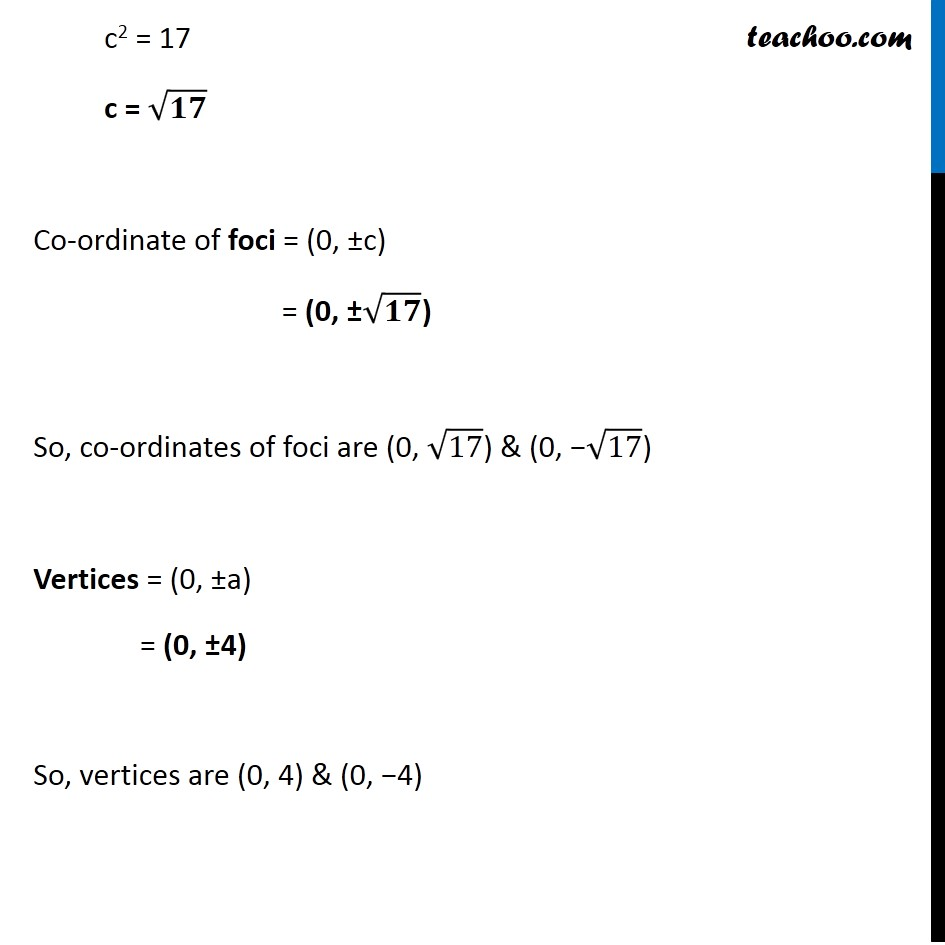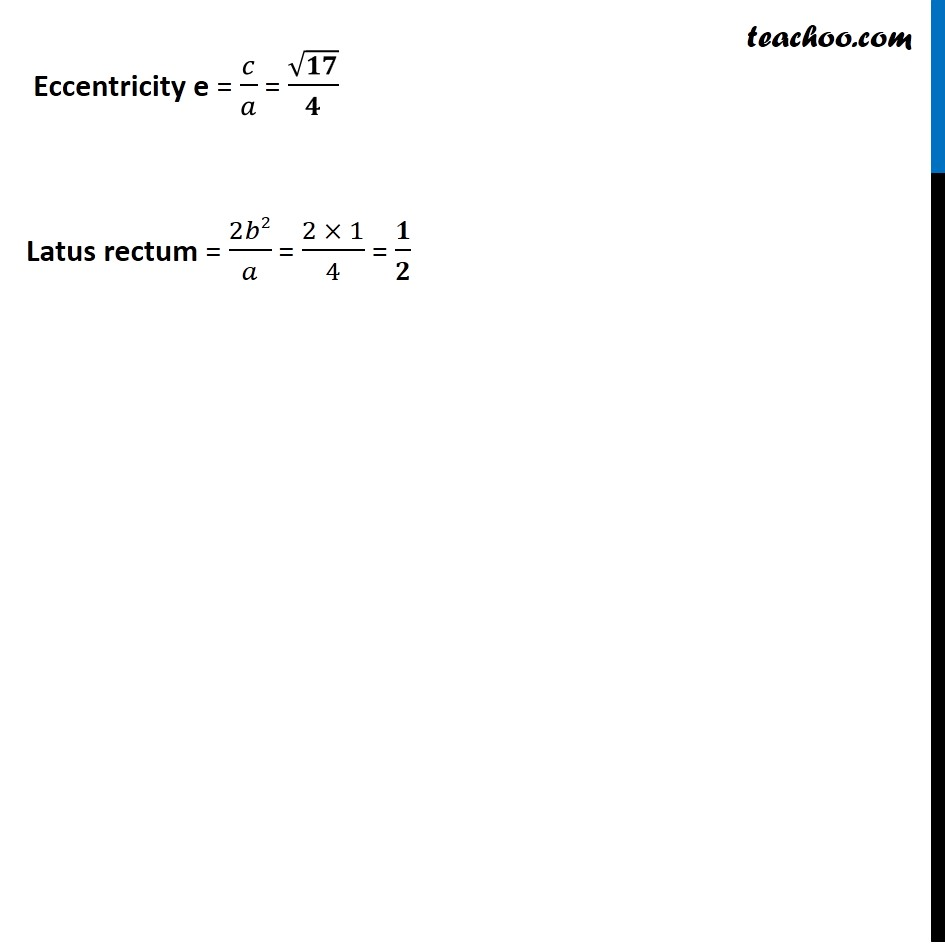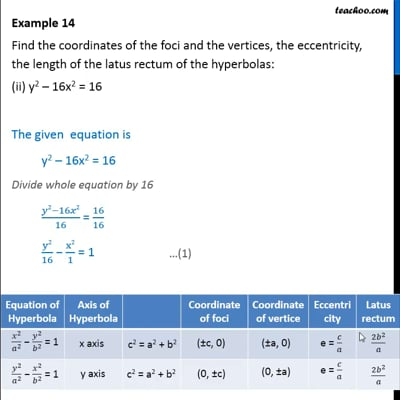This video is only available for Teachoo black users

Introducing your new favourite teacher - Teachoo Black, at only ₹83 per month

### Transcript

Example 14 Find the coordinates of the foci and the vertices, the eccentricity, the length of the latus rectum of the hyperbolas: (ii) y2 – 16x2 = 16 The given equation is y2 – 16x2 = 16 Divide whole equation by 16 (𝑦2−16𝑥2)/16 = 16/16 𝑦2/16 − 𝑥2/1 = 1 The above equation is of the form 𝑦^2/𝑎^2 − 𝑥^2/𝑏^2 = 1 Comparing (1) & (2) a2 = 16 a = 4 & b2 = 1 b = 1 Also , c2 = a2 + b2 c2 = 16 + 1 c2 = 17 c = √𝟏𝟕 Co-ordinate of foci = (0, ±c) = (0, ±√𝟏𝟕) So, co-ordinates of foci are (0, √17) & (0, −√17) Vertices = (0, ±a) = (0, ±4) So, vertices are (0, 4) & (0, −4) Eccentricity e = 𝑐/𝑎 = √𝟏𝟕/𝟒 Latus rectum = 2𝑏2/𝑎 = (2 × 1)/4 = 𝟏/𝟐